Next: 7.4 Ring Oscillator Up: 7. Case Studies Previous: 7.2 CMOS Inverter

Subsections

# 7.3 6T SRAM Cell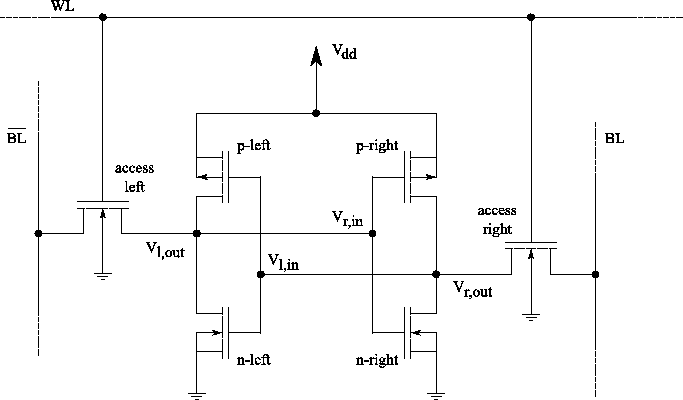Static random access memory (SRAM) can retain its stored information as long as power is supplied. This is in contrast to dynamic RAM (DRAM) where periodic refreshes are necessary or non-volatile memory where no power needs to be supplied for data retention, as for example flash memory. The term random access'' means that in an array of SRAM cells each cell can be read or written in any order, no matter which cell was last accessed.

The structure of a 6 transistor SRAM cell, storing one bit of information, can be seen in Figure 7.18. The core of the cell is formed by two CMOS inverters, where the output potential of each inverter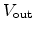is fed as input into the other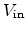. This feedback loop stabilizes the inverters to their respective state.

The access transistors and the word and bit lines, WL and BL, are used to read and write from or to the cell. In standby mode the word line is low, turning the access transistors off. In this state the inverters are in complementary state. When the p-channel MOSFET of the left inverter is turned on, the potential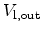is high and the p-channel MOSFET of inverter two is turned off,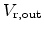is low.

To write information the data is imposed on the bit line and the inverse data on the inverse bit line,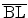. Then the access transistors are turned on by setting the word line to high. As the driver of the bit lines is much stronger it can assert the inverter transistors. As soon as the information is stored in the inverters, the access transistors can be turned off and the information in the inverter is preserved.

For reading the word line is turned on to activate the access transistors while the information is sensed at the bit lines.

## 7.3.1 Static Noise Margin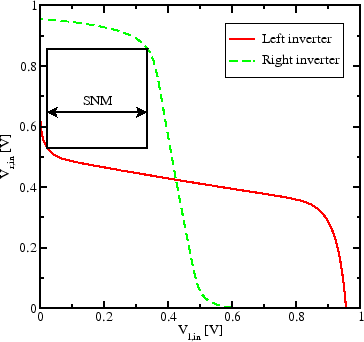A key figure of merit for an SRAM cell is its static noise margin (SNM). It can be extracted by nesting the largest possible square in the two voltage transfer curves (VTC) of the involved CMOS inverters, as seen in Figure 7.19. The SNM is defined as the side-length of the square, given in volts. When an external DC noise is larger than the SNM, the state of the SRAM cell can change and data is lost.

The effect of NBTI impacts one or both p-channel MOSFETs in the SRAM cell, depending on the charge state during temperature stress. The result is a degraded VTC and, therefore, a degraded SNM.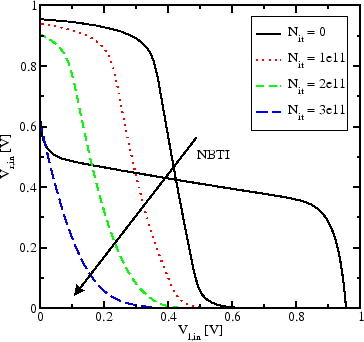Figure 7.20 shows the impact of NBTI on an SRAM cell. Here, only one of the p-channel MOSFETs is degraded. This is the typical situation when the information stored in the cell does not change frequently. An interface trap density of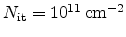is enough to drastically reduce the SNM. At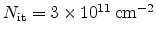the window completely disappears and the SRAM cell loses its functionality.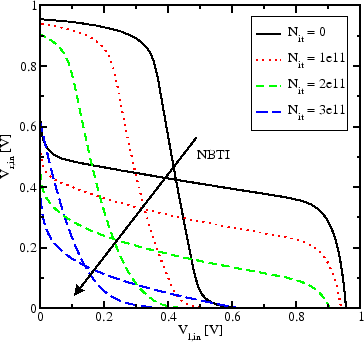Assumed degradation of both p-channel MOSFETs results in shift of the VTCs of both inverters (Figure 7.21). This leads to a complete degeneration of the original VTCs.Next: 7.4 Ring Oscillator Up: 7. Case Studies Previous: 7.2 CMOS Inverter

R. Entner: Modeling and Simulation of Negative Bias Temperature Instability